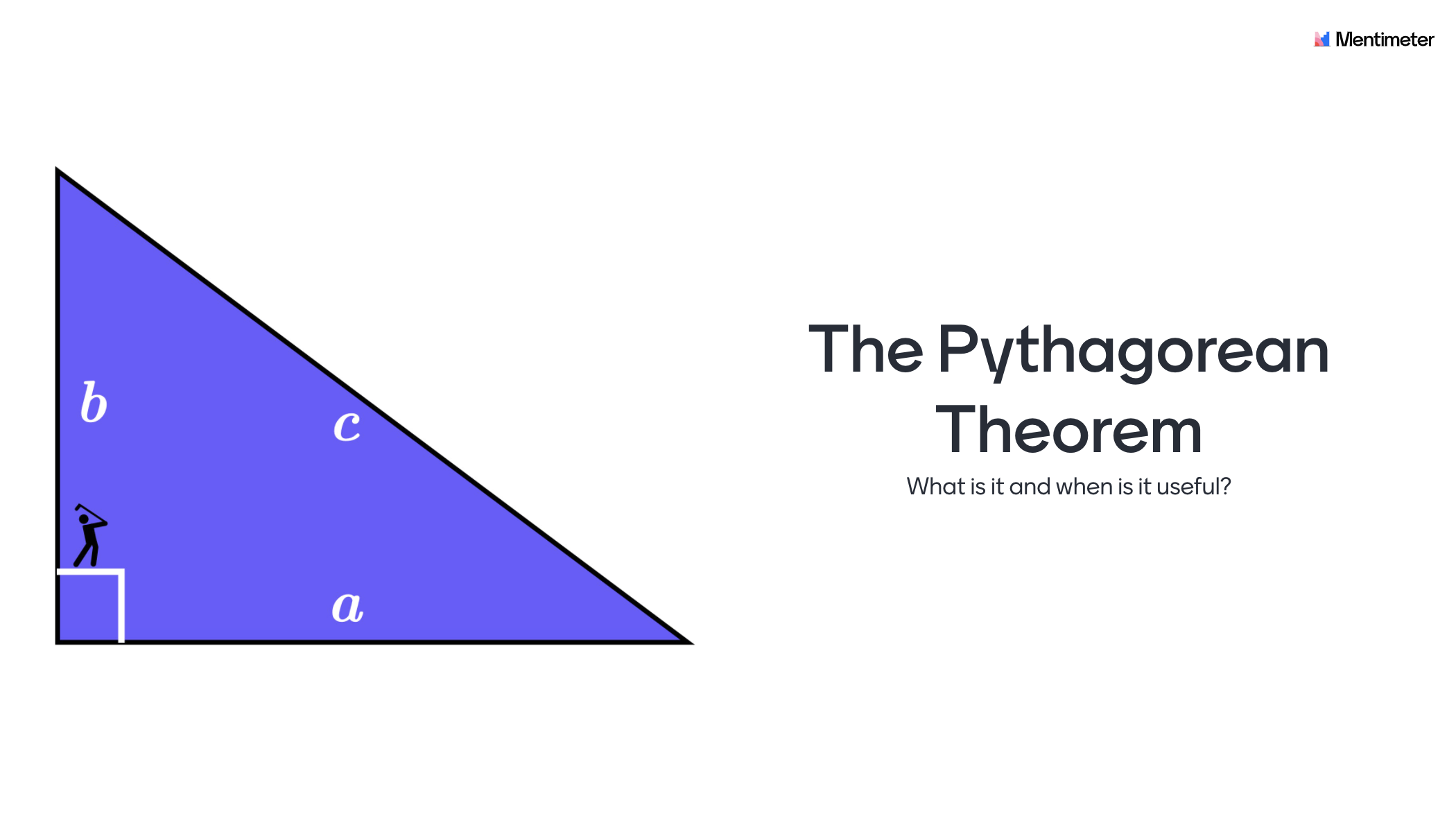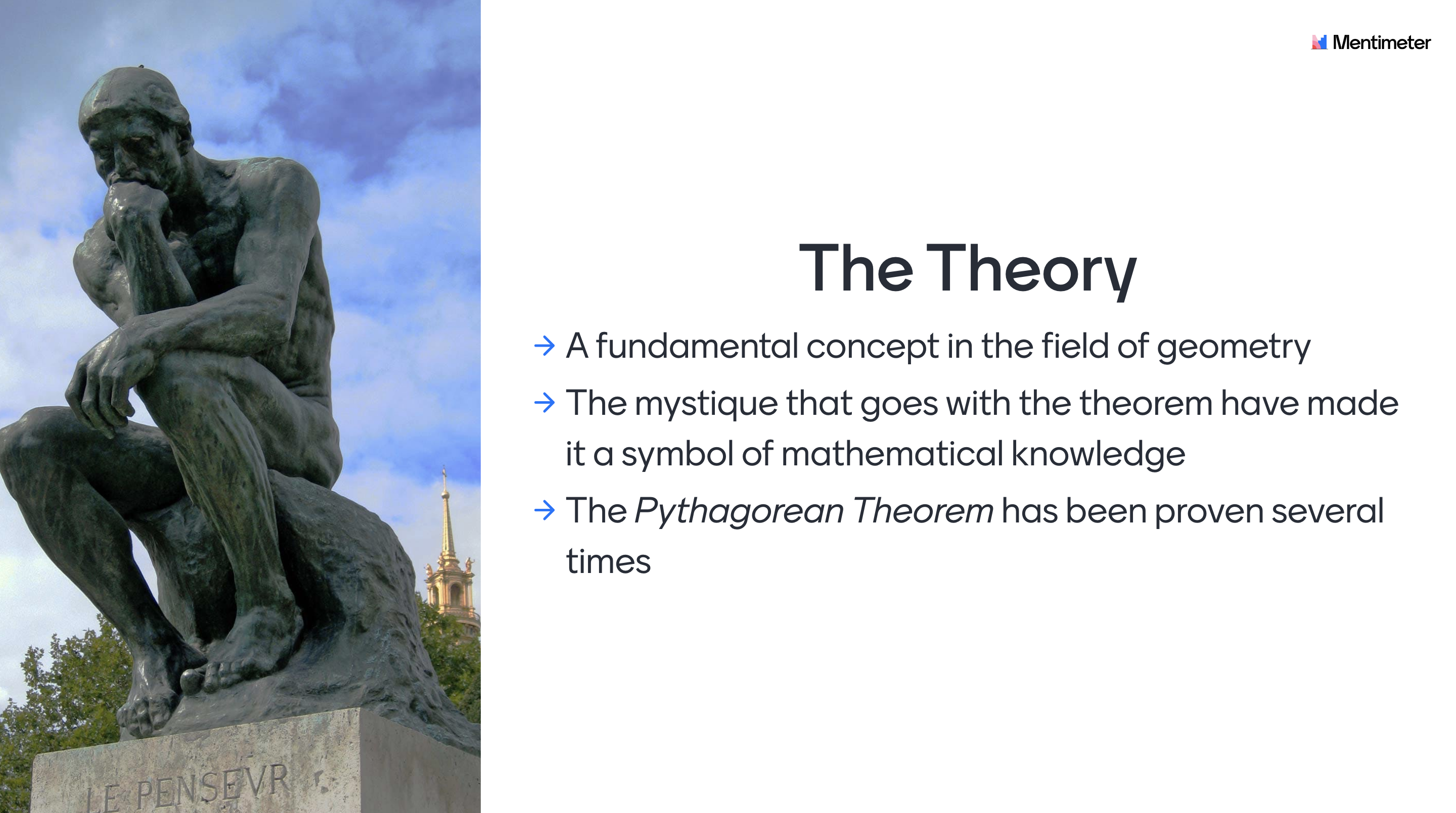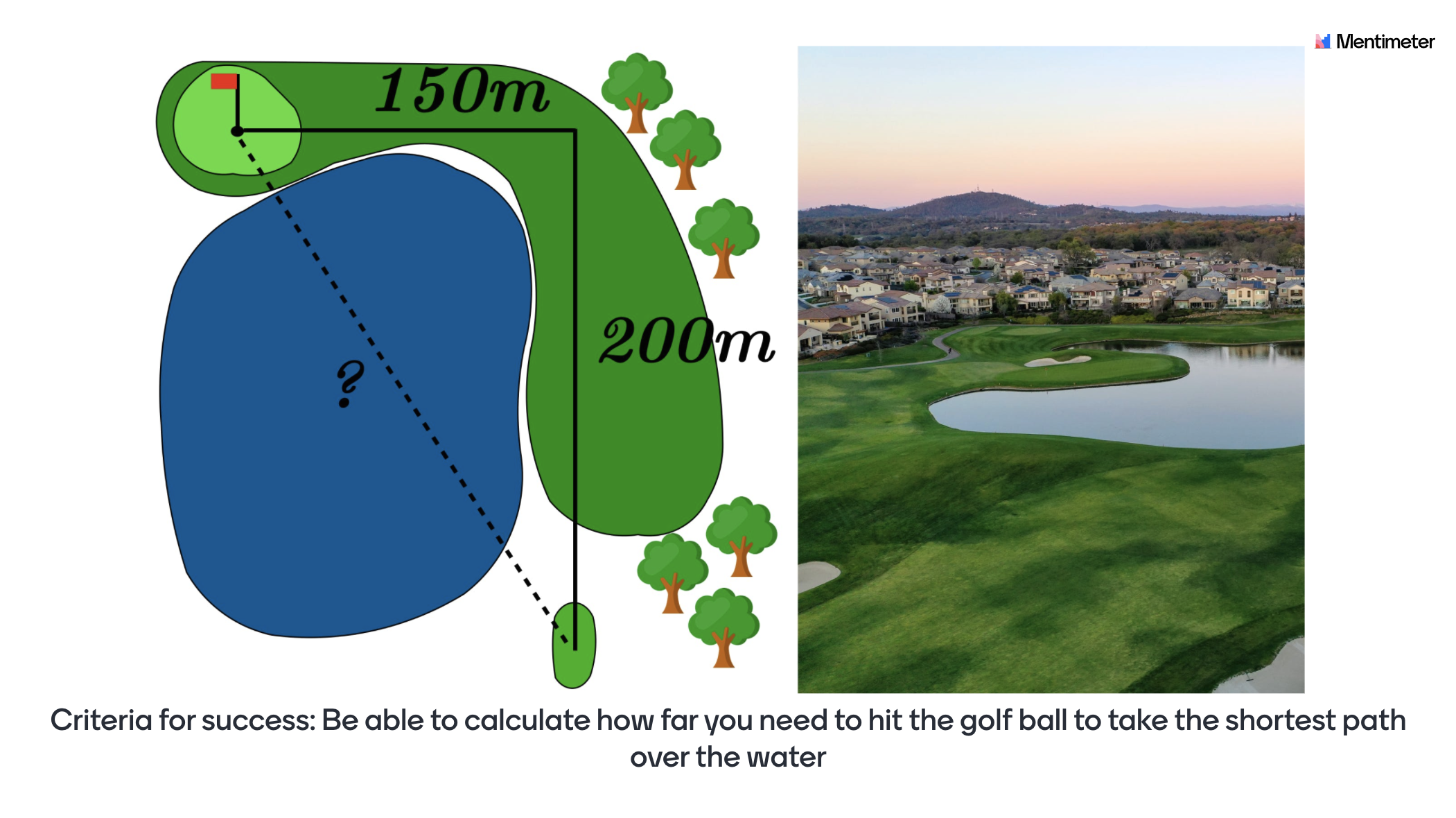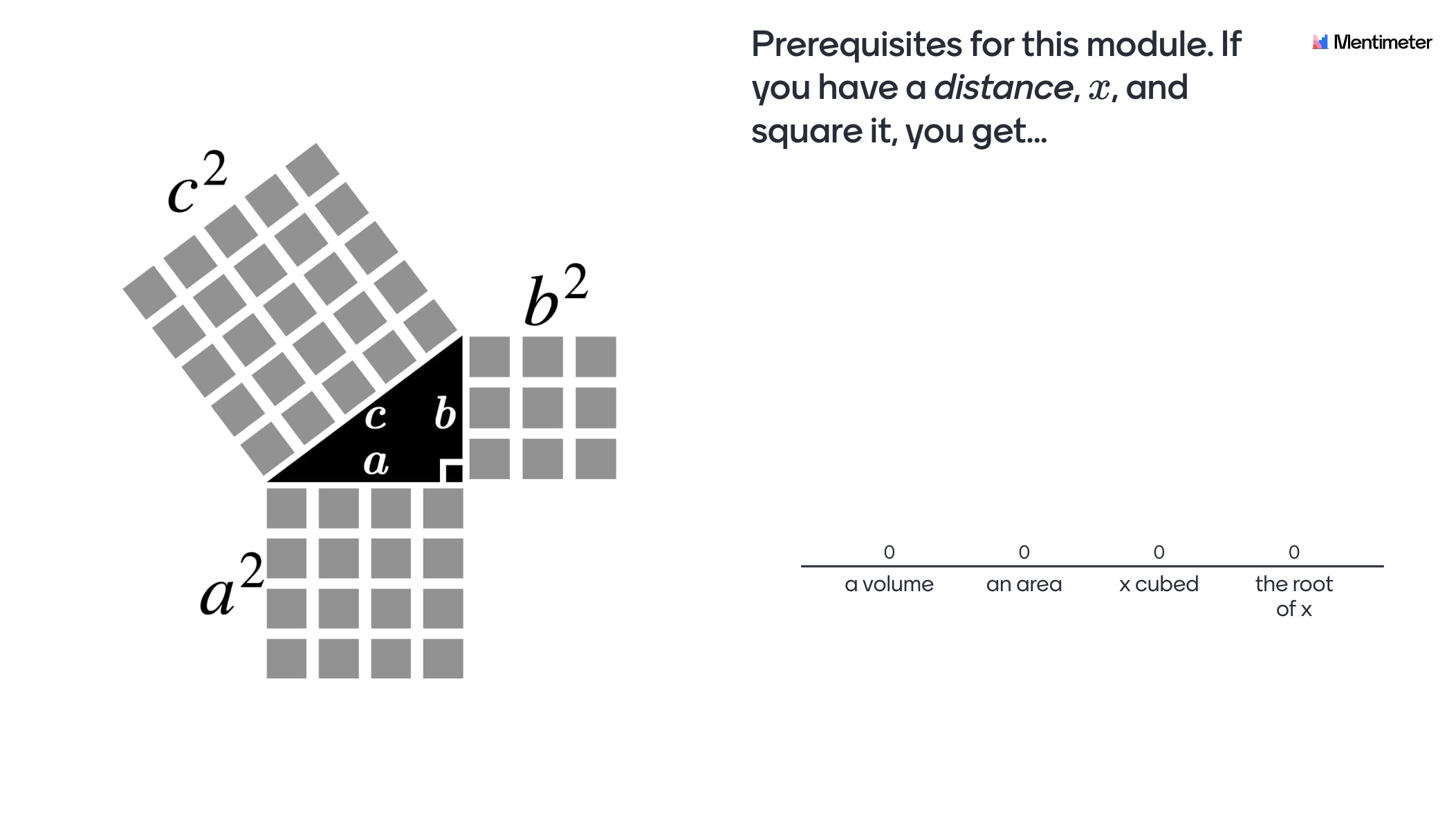# The Pythagorean theorem

This Mentimeter template guides your students through an introduction to the Pythagorean theorem. The template is designed primarily as an introduction but can also be used in the middle or as a form of reinforcement at the end of a subject block on geometry.## How to use the template

The students need a pencil and paper for some of the questions in the presentation.

Here follows some ideas on how the slides are intended to be used but feel free to use the template however you like. Included in each cell is the purpose and other information that could be of use for you as a teacher. A general idea regarding all questions is that the students should work after the model Think-Pair-Share (TPS). When implementing TPS the students should first answer the question individually, then discuss in pairs (or groups of three) followed by a classroom discussion. As a concluding remark. The content in the slides are designed primarily for developing the students understanding of mathematical concepts rather than the procedural 'know how'. Therefore discussions and questions from the students is a central part of using the presentation where your expertise as a teacher is central to clarify misconceptions and lead the classroom discussions.## Theoretical background

The Pythagorean theorem has been proven several times by adapting different mathematical methods and could be considered one of the most fundamental relations in the field of geometry. Some of the proofs that have been given dates back thousands of years and the mystique that goes with the theorem have made it a symbol of mathematical knowledge.

The theorem have never been assigned with a place and time for its discovery but are rather believed to have been discovered on multiple occasions and over a larger geographical area. Even though it carries the name of the greek philosopher Pythagoras who was active around 500 years before Christ the Old Babylonians had knowledge about the Pythagorean triples a millennia earlier. Today students at high school and upper elementary school are probably most familiar with the right-angled representation and the proof that is associated with it. An explanation could be that the Pythagorean theorem is first displayed in geometry class but the depth of knowledge that surrounds it goes beyond.Slide 1 - Introduction: Introduce the theme of the lecture and the learning goals. For example you could mention that the Pythagorean theorem is central in geometry and could be seen as an introduction to the 'distance formula' between any two points in the coordinate system.

Slide 2 - General information: Inform the students regarding the TPS methodology and how they can access the digital lecture.

Slide 3 - Criteria for success: Introduce a "criteria for success" for the lesson with a problem that will be revisited and solved at the end of the lesson. The idea here is to make the learning visible, so the students can see for themselves that they’ve actually learned something.

Slide 4 - What is "Pythagoras"?: By giving some background information about the name of the theorem, the students have a better chance of getting a solid memory of it.

Slide 5 - What is a theorem?: The idea here is that the student should start to reason about what a "theorem" is. A theorem is a central concept in mathematics, and this could be the first time students are introduced to this concept.Slide 6 - A theorem is…: Here a brief definition of a theorem is presented. Here you could mention that theorems will be very important if you want to study higher level mathematics.

Slide 7 - Prerequisites for understanding the rest of the presentation: The concept of squaring and taking the square root is central to understanding the visual intuition of the Pythagorean theorem.

Slide 8 - Definition for a right-angled triangle: The purpose of this slide is to give the students a visual representation of where the hypotenuse and the cathetus are located in a right-angled triangle.

Slide 9 - Create a relation: This is a key slide. The idea here is to get the students to discover the Pythagorean theorem, instead of just giving them the formula.  The pupils should elaborate with squaring, square rooting, adding and subtracting in order to try finding a relationship e.g.: 3² + 4² = 5², 5² - 4² = 3² or 5² + 3² = 4² (or some version of a² + b² = c²)

Slide 10 - Did you find the relation?: This is a key slide and a continuation from slide 9. The purpose of this slide is that the students should start to understand that the sum of the a2-cubes with the b2-cubes adds up to the total amount of the c2-cubes. It symbolizes a visual proof of the Pythagorean theorem.Slide 11 - What is (a2 + b2)(1/2)?: This is a key slide. The idea is that a visual representation of the right-angled triangle with the squared sides should facilitate the understanding of the Pythagorean theorem. Here is a good opportunity to see if the students have understood that square-rooting the expression (a² + b²) gives the length of the hypotenuse.

Slide 12 - Criteria for success revisited: Return to the problem that was introduced in the beginning of the lecture to make the students realize they’ve actually learnt something.

Slide 13 - Q&A: Let the students individually write down what they still feel is unclear about the pythagorean theorem.

Slide 14-20 - Quiz Competition!: Ask the student to enter their real names. Let the students  compete in a fun way while you as a teacher can get a sense of the individual level of knowledge. This information could be used to give support for students in need of guidance.